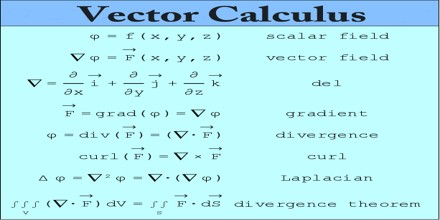Vector Calculus

Definition

Vector Calculus (or vector analysis) is closely linked with the needs of physics and mechanics. It is a field of mathematics concerned with multivariate real analysis of vectors in an inner product space of two or more dimensions; some results are those that involve the cross product can only be applied to three dimensions. It consists of a suite of formulae and problem solving techniques very useful for engineering and physics.Only in the middle of the 19th century, by the endeavors of a number of scientists, was a vector calculus created in which operations were carried out directly on vectors, without resorting to their coordinate representations. The fundamentals of vector calculus were laid by the British mathematician W. Hamilton and the German mathematician G. Grassmann in their work on hypercomplex numbers (1844-50). In the conventional form using cross products, vector calculus does not generalize to higher dimensions, while the alternative approach of geometric algebra, which uses exterior products, does generalize.

Vector Calculus is concerned with scalar fields, which associate a scalar to every point in space, and vector fields, which associate a vector to every point in space. For example, the temperature of a swimming pool is a scalar field: to each point we associate a scalar value of temperature. The water flow in the same pool is a vector field: to each point we associate a velocity vector. Vector fields are often used to model, for example, the speed and direction of a moving fluid throughout space, or the strength and direction of some force, such as the magnetic or gravitational force, as it changes from point to point.Vector Algebra

An important role in vector algebra is played by linear operations on vectors: adding vectors and multiplying them by real numbers. The sum a + b of vectors a and b is the vector extending from the beginning of vector a to the end of vector b such that the beginning of vector b is joined to the end of vector a. The product α a of vector a and the number α is a vector that is collinear with vector a and has a length |α|· |a| and a direction that coincides with the direction of a when α> 0 and is opposite to that of a when α< 0. Vector -1 · a is the inverse vector of a and is denoted by -a.

Applications of Vector Calculus

For a continuously differentiable function of several real variables, a point P, that is a set of values for the input variables, which is viewed as a point in Rn, which is critical if all of the partial derivatives of the function are zero at P, or, equivalently, if it’s gradient is zero. The critical values are the values of the function at the critical points.Vectors sounds are complicated, but they are common when giving directions. For example, telling someone to walk to the end of a street before turning left and walking five more blocks is an example of using vectors to give directions.

Navigating by air and by boat is generally done using vectors. Planes are given a vector to travel, and they use their speed to determine how far they need to go before turning or landing. Flight plans are made using a series of vectors.

Sports instructions are based on using vectors. Wide receivers playing American football, for example, might run a route where they run seven yards down the field before turning left 45 degrees and running in that direction. Sports commentary also depends on vectors. Only a few sports have fields with grids, so discussions revolve around the direction and speed of the player.